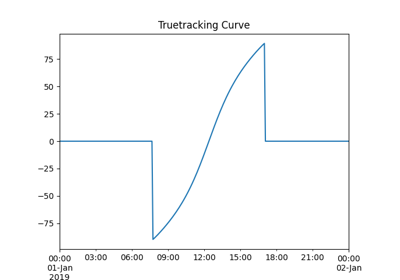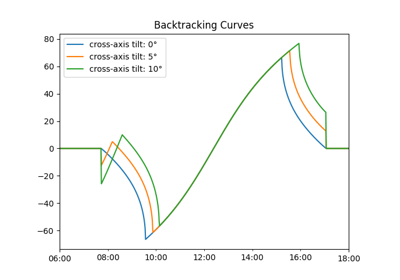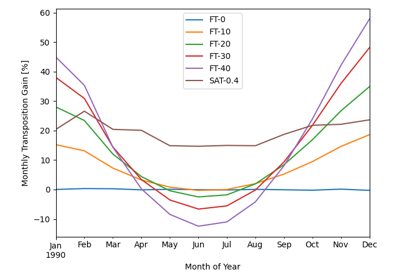# pvlib.tracking.singleaxis¶

pvlib.tracking.singleaxis(apparent_zenith, apparent_azimuth, axis_tilt=0, axis_azimuth=0, max_angle=90, backtrack=True, gcr=0.2857142857142857, cross_axis_tilt=0)[source]

Determine the rotation angle of a single-axis tracker when given particular solar zenith and azimuth angles.

See 1 for details about the equations. Backtracking may be specified, and if so, a ground coverage ratio is required.

Rotation angle is determined in a right-handed coordinate system. The tracker axis_azimuth defines the positive y-axis, the positive x-axis is 90 degrees clockwise from the y-axis and parallel to the Earth’s surface, and the positive z-axis is normal to both x & y-axes and oriented skyward. Rotation angle tracker_theta is a right-handed rotation around the y-axis in the x, y, z coordinate system and indicates tracker position relative to horizontal. For example, if tracker axis_azimuth is 180 (oriented south) and axis_tilt is zero, then a tracker_theta of zero is horizontal, a tracker_theta of 30 degrees is a rotation of 30 degrees towards the west, and a tracker_theta of -90 degrees is a rotation to the vertical plane facing east.

Parameters
• apparent_zenith (float, 1d array, or Series) – Solar apparent zenith angles in decimal degrees.

• apparent_azimuth (float, 1d array, or Series) – Solar apparent azimuth angles in decimal degrees.

• axis_tilt (float, default 0) – The tilt of the axis of rotation (i.e, the y-axis defined by axis_azimuth) with respect to horizontal, in decimal degrees.

• axis_azimuth (float, default 0) – A value denoting the compass direction along which the axis of rotation lies. Measured in decimal degrees east of north.

• max_angle (float, default 90) – A value denoting the maximum rotation angle, in decimal degrees, of the one-axis tracker from its horizontal position (horizontal if axis_tilt = 0). A max_angle of 90 degrees allows the tracker to rotate to a vertical position to point the panel towards a horizon. max_angle of 180 degrees allows for full rotation.

• backtrack (bool, default True) – Controls whether the tracker has the capability to “backtrack” to avoid row-to-row shading. False denotes no backtrack capability. True denotes backtrack capability.

• gcr (float, default 2.0/7.0) – A value denoting the ground coverage ratio of a tracker system which utilizes backtracking; i.e. the ratio between the PV array surface area to total ground area. A tracker system with modules 2 meters wide, centered on the tracking axis, with 6 meters between the tracking axes has a gcr of 2/6=0.333. If gcr is not provided, a gcr of 2/7 is default. gcr must be <=1.

• cross_axis_tilt (float, default 0.0) – The angle, relative to horizontal, of the line formed by the intersection between the slope containing the tracker axes and a plane perpendicular to the tracker axes. Cross-axis tilt should be specified using a right-handed convention. For example, trackers with axis azimuth of 180 degrees (heading south) will have a negative cross-axis tilt if the tracker axes plane slopes down to the east and positive cross-axis tilt if the tracker axes plane slopes up to the east. Use calc_cross_axis_tilt() to calculate cross_axis_tilt. [degrees]

Returns

dict or DataFrame with the following columns

• tracker_theta: The rotation angle of the tracker. tracker_theta = 0 is horizontal, and positive rotation angles are clockwise. [degrees]

• aoi: The angle-of-incidence of direct irradiance onto the rotated panel surface. [degrees]

• surface_tilt: The angle between the panel surface and the earth surface, accounting for panel rotation. [degrees]

• surface_azimuth: The azimuth of the rotated panel, determined by projecting the vector normal to the panel’s surface to the earth’s surface. [degrees]

References

1

Kevin Anderson and Mark Mikofski, “Slope-Aware Backtracking for Single-Axis Trackers”, Technical Report NREL/TP-5K00-76626, July 2020. https://www.nrel.gov/docs/fy20osti/76626.pdf

## Examples using pvlib.tracking.singleaxis¶